## 分类预测与scikit-learn

Posted on Jun 6, 2018

# 分类任务介绍

• 预测某个人会不会得癌症
• 识别图片的内容是哪个字母或者数字

## 多分类

• 一对其余（One-versus-Rest，简称OvR，或者One-versus-all，简称OvA）
• 一对一（One-versus-One，简称 OvO）

• 0 vs. 1
• 0 vs. 2
• 1 vs. 0
• 1 vs. 2
• 9 vs. 7
• 9 vs. 8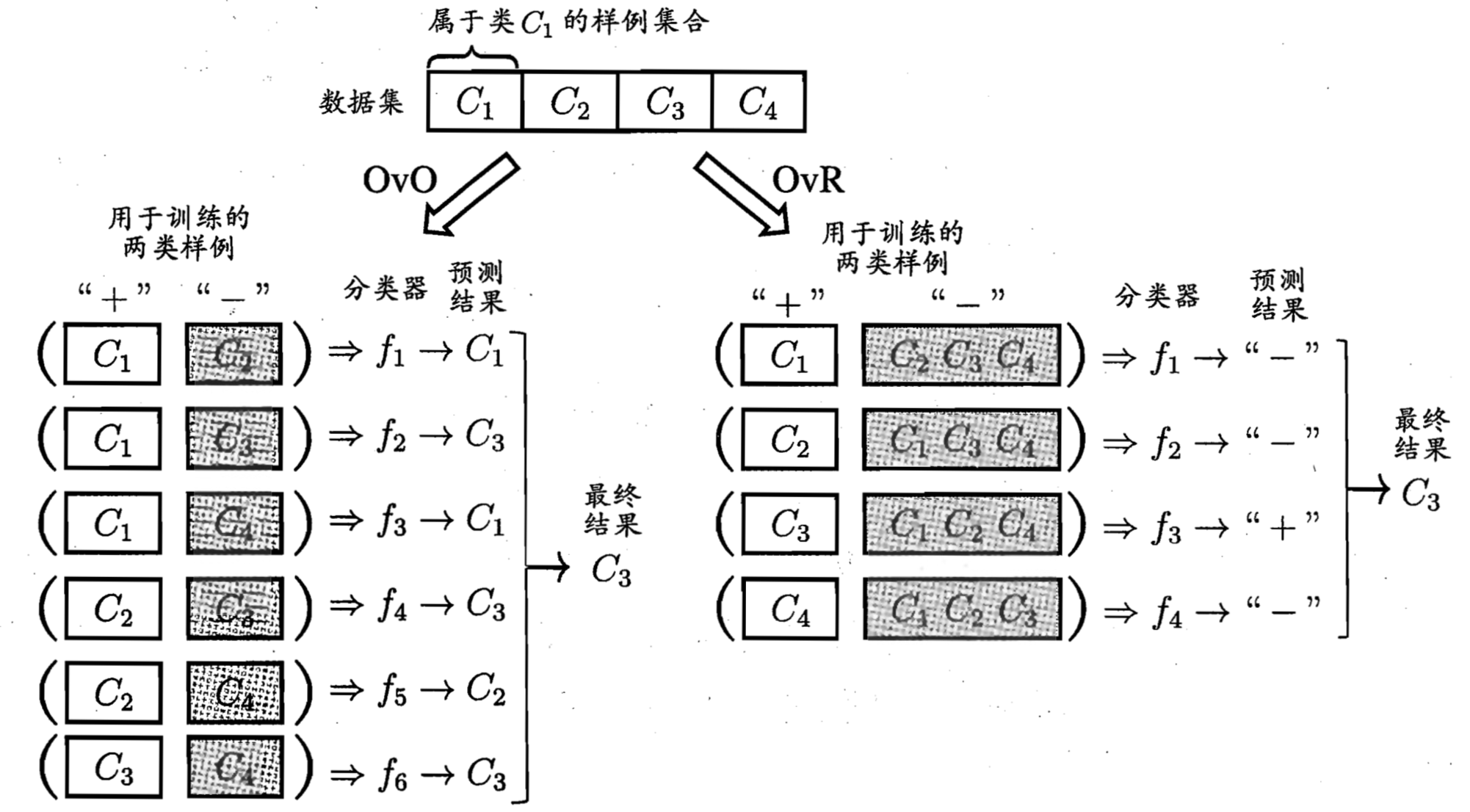# 实验描述

A B C D E F G H I J K
1000025 5 1 1 1 2 1 3 1 1 2
1002945 5 4 4 5 7 10 3 2 1 2
1015425 3 1 1 1 2 2 3 1 1 2
1016277 6 8 8 1 3 4 3 7 1 2
1017023 4 1 1 3 2 1 3 1 1 2
1017122 8 10 10 8 7 10 9 7 1 4
1018099 1 1 1 1 2 10 3 1 1 2
1018561 2 1 2 1 2 1 3 1 1 2
1033078 2 1 1 1 2 1 1 1 5 2
1033078 4 2 1 1 2 1 2 1 1 2
1035283 1 1 1 1 1 1 3 1 1 2
1036172 2 1 1 1 2 1 2 1 1 2
1041801 5 3 3 3 2 3 4 4 1 4
1043999 1 1 1 1 2 3 3 1 1 2
1044572 8 7 5 10 7 9 5 5 4 4
1047630 7 4 6 4 6 1 4 3 1 4
1048672 4 1 1 1 2 1 2 1 1 2
1049815 4 1 1 1 2 1 3 1 1 2
1050670 10 7 7 6 4 10 4 1 2 4
1050718 6 1 1 1 2 1 3 1 1 2

A Sample code number Number
B Clump Thickness 1 - 10
C Uniformity of Cell Size 1 - 10
D Uniformity of Cell Shape 1 - 10
E Marginal Adhesion 1 - 10
F Single Epithelial Cell Size 1 - 10
G Bare Nuclei 1 - 10
H Bland Chromatin 1 - 10
I Normal Nucleoli 1 - 10
J Mitoses 1 - 10
K Class 2：良性；4：恶性

• 2 表示良性肿瘤
• 4 表示恶性肿瘤

## 实验环境

• Apple OS X 10.13
• Python 3.6.3
• scikit-learn 0.19.1
• matplotlib 2.1.1
• numpy 1.13.3
• graphviz 0.8.3

## 实验数据

# lession2_classification.py

import matplotlib.pyplot as plt
import numpy as np
import pandas as pd

from sklearn.preprocessing import Imputer

def prepare_data():
names=['id', 'clump_thickness', 'cell_size', 'cell_shape',
'bland_chromatin', 'normal_nucleoli', 'mitoses', 'class'])
input_data = input_data.apply(pd.to_numeric, errors='coerce')

imputer = Imputer(strategy="mean")
X = imputer.fit_transform(input_data)
return pd.DataFrame(X, columns=input_data.columns)


# lession2_classification.py

from sklearn.model_selection import train_test_split

def data_split(input_data):
target = input_data['class'].copy()
return input_data.drop(['class'], axis=1), target

if __name__ == '__main__':
input_data = prepare_data()

train_set, test_set = train_test_split(input_data,
test_size=0.1, random_state=59)
train_data, train_value = data_split(train_set)
test_data, test_value = data_split(test_set)


• train_data：训练集的数据
• train_value：训练集的结果
• test_data：测试集的数据
• test_value：测试集的结果

# 性能度量

## 迷惑矩阵与精度

Cat Dog Rabbit
Cat 5 2 0
Dog 3 3 2
Rabbit 0 1 11

• 有7（5+2）个用例被分类为Cat，其中有2个是错误的，它们的真实分类是Dog。
• 有8（3+3+2）个用例被分类为Dog，其中有5个是错误的，其中有3个是Cat，有2个是Rabbit。
• 有12（1+11）个用例别分类为Rabbit，其中有1个用例是错误的，它的真实分类是Dog。

$精度 = 1 - 错误率$

## 查准率与查全率

$P = \frac{TP}{(TP + FP)}$

$R = \frac{TP}{(TP + FN)}$

### F1值

$F1 = \frac{2}{\frac{1}{recall} + \frac{1}{precision}} = 2 * \frac{precision * recall}{precision + recall}$

Classification metrics 中包含了用来对分类器进行性能度量的API。

# lession2_classification.py

import pandas as pd

from sklearn.preprocessing import LabelBinarizer
from sklearn.metrics import f1_score
from sklearn.metrics import precision_score
from sklearn.metrics import recall_score

def measure_predict(y_true, y_predict):
lb = LabelBinarizer()
bin_true = pd.Series(lb.fit_transform(y_true)[:,0])
bin_predict = pd.Series(lb.fit_transform(y_predict)[:,0])

print("confusion matrix: \n{}\n".format(
confusion_matrix(bin_true, bin_predict)))
print("recall_score: {}\n".format(
recall_score(bin_true, bin_predict)))
print("precision_score: {}\n".format(
precision_score(bin_true, bin_predict)))
print("f1_score : {}\n".format(
f1_score(bin_true, bin_predict)))


• y_true：真实的结果
• y_predict：算法预测的结果

# 对数回归

• 如果 z < 0, y = 0
• 如果 z > 0, y = 1

（如果z=0，我们可以任意规定y取0或者1。）

$y = \frac{1}{1+e^{-z}}$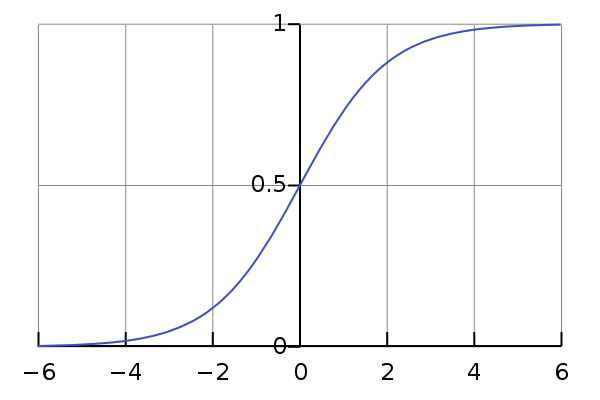$y = \frac{1}{1+e^{-(w^{T}x+b)}}$

## scikit-learn API

# lession2_classification.py

from sklearn.linear_model import LogisticRegression

def logistic_reg(train_data, train_value, test_data):
logReg = LogisticRegression()
logReg.fit(train_data, train_value)
return logReg.predict(test_data)


# 决策树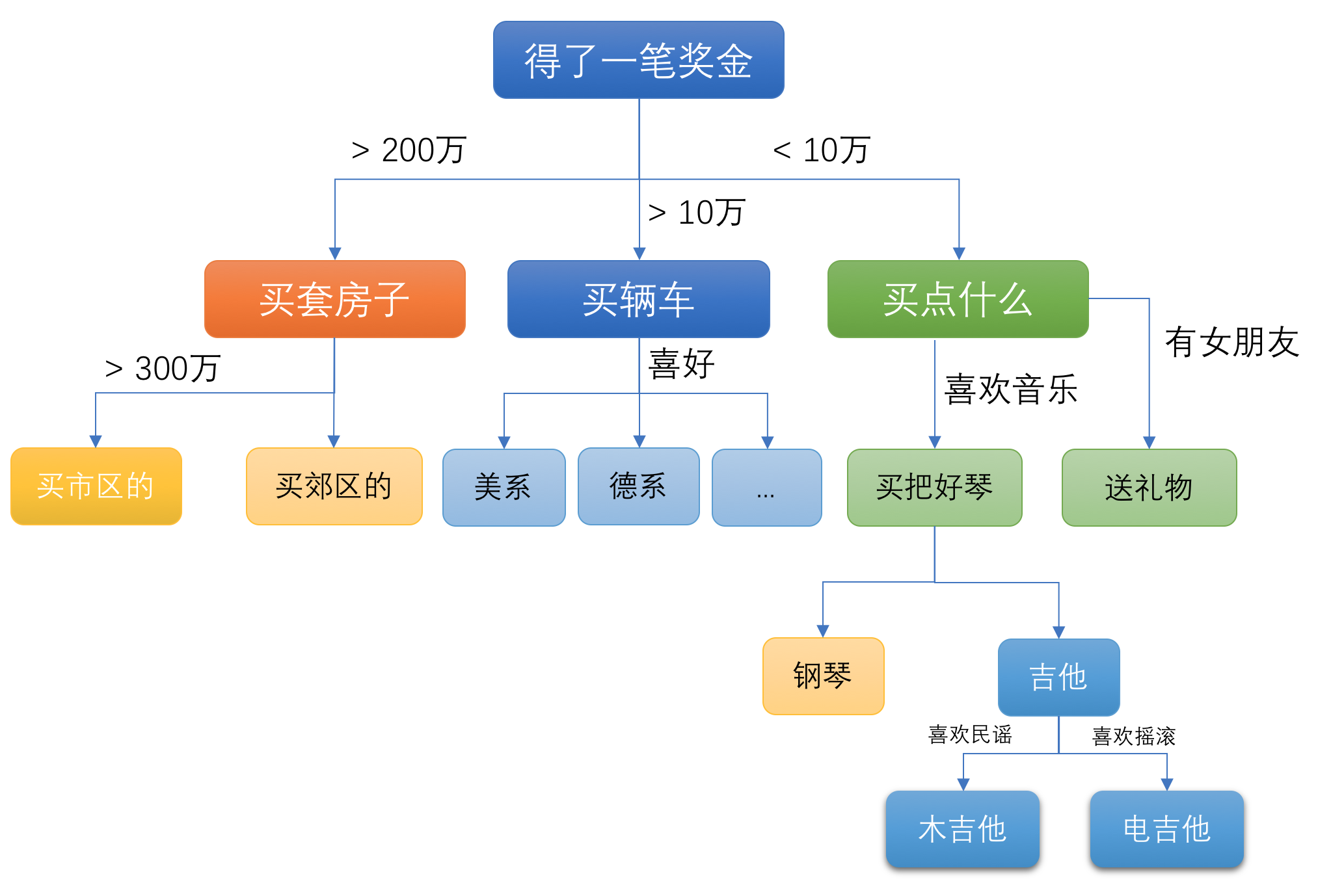## 熵与相对熵（信息增益）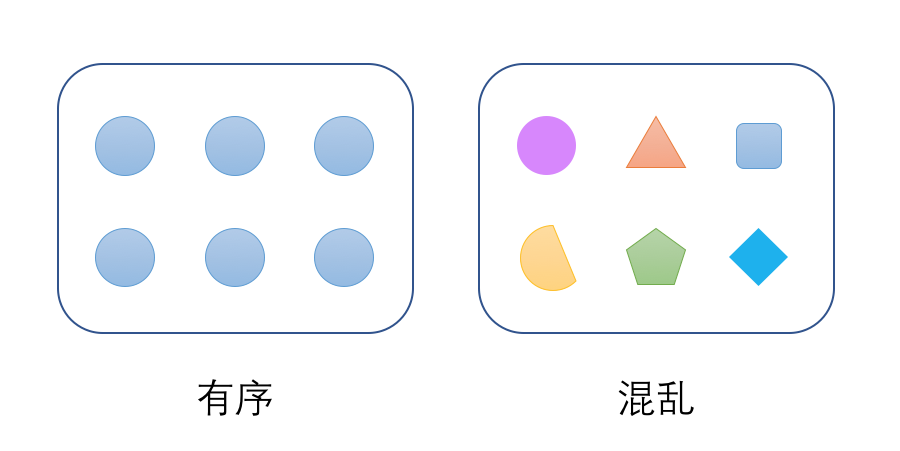$Ent(D) = - \sum_{k=1}^{n}p_{k}log_{b}p_{k}$

• n是集合中数据分类的总数。例如：性别的分类只有2种。
• $p_k$是第k种数据出现的概率。例如：50%或者95%。

$Ent = -1log_{2}1 = 0$

$Ent(D1) = - \sum_{k=1}^{2}p_{k}log_{2}p_{k} = - (0.5* log_{2}0.5 + 0.5* log_{2}0.5) = - log_{2}\frac{1}{2} = 1$

$Ent(D2) = - \sum_{k=1}^{2}p_{k}log_{2}p_{k} = - (0.95* log_{2}0.95 + 0.05* log_{2}0.05) = 0.0703 + 0.216 = 0.2863$

$H(D|X) = - \sum_{k=1}^{v}p(D,X)log_{2}p(D|X)$

$相对熵（信息增益） = 熵 - 条件熵$

## CART算法与基尼不纯度

CART算法是基于基尼不纯度（Gini impurity）为基础来构建决策树。Gini不纯度描述了集合数据的不一致性，和熵表达的概念是类似，这个值越大表示数据越不一致，或者说越不均匀。

$G = \sum_{k=1}^n\sum_{k'≠k}p_{k}p_{k'} =1- \sum_{k=1}^{n}p_{k,k'}^2$

$Gini\_index(D, a) = \sum_{v=1}^V\frac{D^v}{D}Gini(D^v)$

### scikit-learn API

# lession2_classification.py

from sklearn.tree import DecisionTreeClassifier

def dicision_tree(train_data, train_value, test_data):
tree_clf = DecisionTreeClassifier(max_depth=5)
tree_clf.fit(train_data, train_value)
write_file(tree_clf)
return tree_clf.predict(test_data)



### 可视化

# lession2_classification.py

import graphviz

from sklearn.tree import export_graphviz

def write_file(tree_clf):
dot_data = export_graphviz(tree_clf, out_file=None,
filled=True, proportion=True, rounded=True)
graph = graphviz.Source(source=dot_data,
filename="./decision_tree", format="png")
graph.render()


pip3 install graphviz
brew install graphviz


export_graphviz(tree_clf, out_file="./dot_data.txt", filled=True, proportion=True, rounded=True)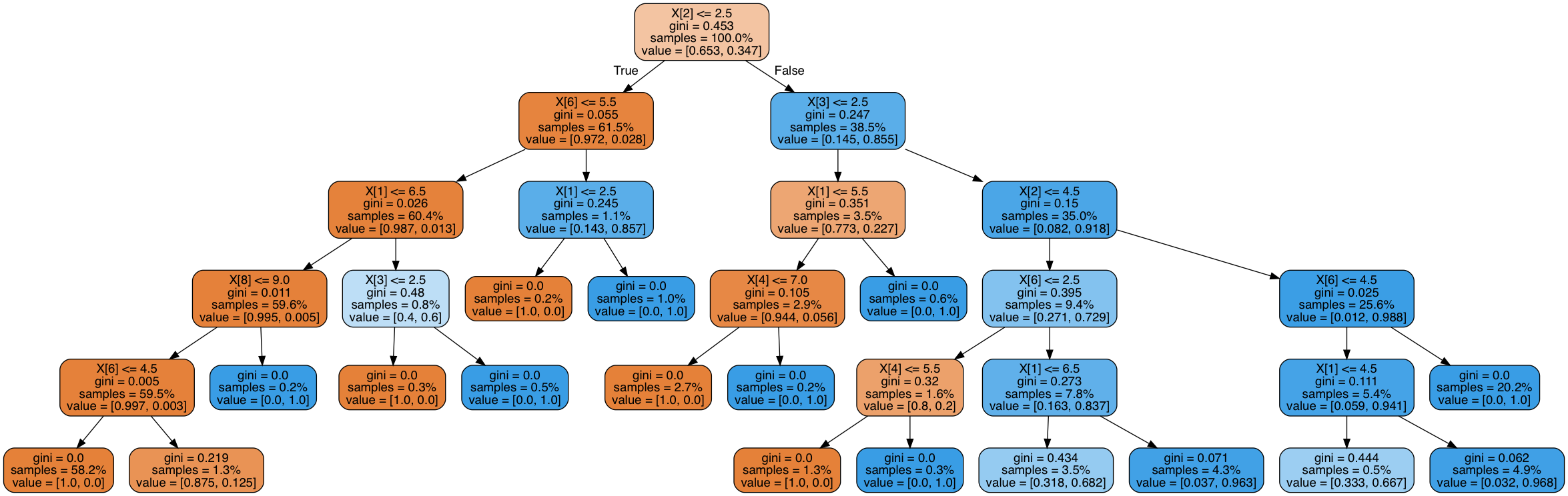# 预测结果

== logistic regression ==
confusion matrix:
[[47  0]
[23  0]]


== decision tree ==
confusion matrix:
[[44  3]
[ 2 21]]

recall_score: 0.9130434782608695

precision_score: 0.875

f1_score : 0.8936170212765957


• 47个反例中误测了三个反例
• 23个正例中错过了两个正例
• 查全率是91.3%（$\frac{21}{21+2}$）
• 查准率是87.5%（$\frac{21}{21+3}$）
• F1得分是0.894

Contents